# Thom space

A topological space associated with a vector (or sphere) bundle or spherical fibration.

Letbe a vector bundle over a CW-complex. Suppose that one is given a Riemannian metric on it, and consider the unit-disc bundle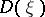associated with. Inlies the unit-sphere subbundle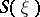; the quotient spaceis the Thom space of the bundle, denoted by. For a compact base space, the Thom space can also be described as the one-point compactification of the total space of the bundle. Moreover, the Thom space is the cone of the projection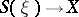and in this way one can define the Thom space of any spherical fibration. Of course, the Thom space is also defined for any bundle with fibre.

Let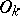be the group of orthogonal transformations of the space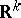. Over its classifying space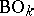there is the-dimensional vector bundle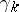, associated with the universal-bundle. The Thom spaceis often denoted byor, and is called the Thom space of the group. Analogously one introduces the Thom spaces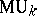,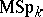, etc., where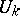and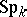are the unitary and symplectic groups, respectively.

The role of the Thom space is to allow one to reduce a series of geometric problems to problems in homotopic topology, and hence to algebraic problems. Thus, the problem of computing a bordism group reduces to the problem of computing a homotopy group of a Thom space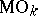,, etc. (cf. , , and also Cobordism). The problem of classifying smooth manifolds reduces to the study of the homotopy properties of the Thom space of the normal bundle (cf. ). The problem of realizing cycles by submanifolds (cf. Steenrod problem) reduces to the study of the cohomology of the Thom spacesand, etc. (see also Transversal mapping; Tubular neighbourhood).

The construction of Thom spaces is natural on the category of bundles: Any morphism of (vector) bundles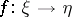induces a continuous mapping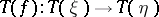. In particular, the Thom space of an-dimensional bundle over a point is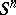, and hence for any-dimensional bundleoverand any pointthere is an inclusion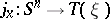(induced by the inclusion of the fibre over). Ifis path connected, then all such inclusions are homotopic, and one can talk about the mapping, which is unique up to homotopy.

For vector bundlesandoverand, respectively, one can define the bundle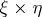over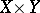. Then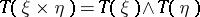(cf. ). In particular, for the trivial bundle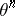one has, whereis the suspension operator, so that. This circumstance allows one to construct spectra of Thom spaces, cf. Thom spectrum.

For a multiplicative generalized cohomology theory(cf. Generalized cohomology theories) there is a pairing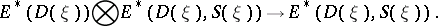There arises a pairingso that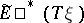is an-module, and this is used in constructing the Thom isomorphism.

The following Atiyah duality theorem is important and often used (cf. , ): Ifis a smooth manifold with boundary(possibly empty) andis its normal bundle, then the Thom spaceis in-duality with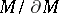.